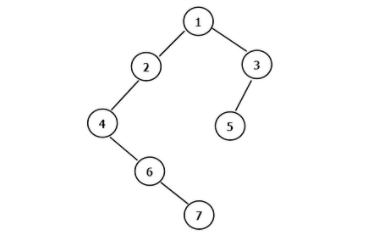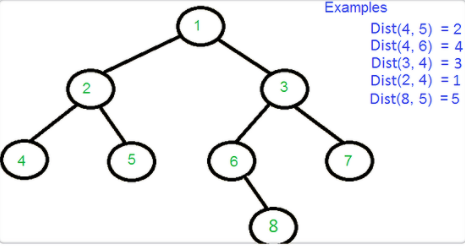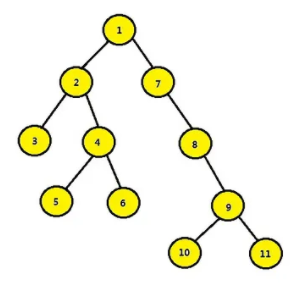[导读]树是数据结构中的重中之重，尤其以各类二叉树为学习的难点。在面试环节中，二叉树也是必考的模块。本文主要讲二叉树操作的相关知识，梳理面试常考的内容。请大家跟随小编一起来复习吧。

(给C语言与CPP编程加星标，提升C/C++技能)1. 前序遍历，中序遍历，后序遍历；
2. 层次遍历；
3. 求树的结点数；
4. 求树的叶子数；
5. 求树的深度；
6. 求二叉树第k层的结点个数；
7. 判断两棵二叉树是否结构相同；
8. 求二叉树的镜像；
9. 求两个结点的最低公共祖先结点；
10. 求任意两结点距离；
11. 找出二叉树中某个结点的所有祖先结点；
12. 不使用递归和栈遍历二叉树；
13. 二叉树前序中序推后序；
14. 判断二叉树是不是完全二叉树；
15. 判断是否是二叉查找树的后序遍历结果；
16. 给定一个二叉查找树中的结点，找出在中序遍历下它的后继和前驱；
17. 二分查找树转化为排序的循环双链表；
18. 有序链表转化为平衡的二分查找树；
19. 判断是否是二叉查找树。

## 1 前序遍历，中序遍历，后序遍历；

### 1.1 前序遍历对于当前结点，先输出该结点，然后输出它的左孩子，最后输出它的右孩子。以上图为例，递归的过程如下：

1. 输出 1，接着左孩子；
2. 输出 2，接着左孩子；
3. 输出 4，左孩子为空，再接着右孩子；
4. 输出 6，左孩子为空，再接着右孩子；
5. 输出 7，左右孩子都为空，此时 2 的左子树全部输出，2 的右子树为空，此时 1 的左子树全部输出，接着 1 的右子树；
6. 输出 3，接着左孩子；
7. 输出 5，左右孩子为空，此时 3 的左子树全部输出，3 的右子树为空，至此 1 的右子树全部输出，结束。

/* 前序遍历递归版 */void PreOrderRec(Node * node){    if (node == nullptr)        return;    cout << node->data << " ";   // 先输出当前结点       PreOrderRec(node->left);     // 然后输出左孩子    PreOrderRec(node->right);    // 最后输出右孩子}/* 前序遍历非递归版 */void PreOrderNonRec(Node * node){    if (node == nullptr)        return;    stack S;    cout << node->data << " ";    S.push(node);    node = node->left;    while (!S.empty() || node)    {        while (node)        {            cout << node->data << " "; // 先输出当前结点              S.push(node);            node = node->left;         // 然后输出左孩子        }                              // while 结束意味着左孩子已经全部输出        node = S.top()->right;         // 最后输出右孩子        S.pop();    }}

### 1.2 中序遍历

1. 1-->2-->4，4 的左孩子为空，输出 4，接着右孩子；
2. 6 的左孩子为空，输出 6，接着右孩子；
3. 7 的左孩子为空，输出 7，右孩子也为空，此时 2 的左子树全部输出，输出 2，2 的右孩子为空，此时 1 的左子树全部输出，输出 1，接着 1 的右孩子；
4. 3-->5，5 左孩子为空，输出 5，右孩子也为空，此时 3 的左子树全部输出，而 3 的右孩子为空，至此 1 的右子树全部输出，结束。
/* 中序遍历递归版 */void InOrderRec(Node * node){    if (node == nullptr)        return;    InOrderRec(node->left);     // 先输出左孩子    cout << node->data << " ";  // 然后输出当前结点    InOrderRec(node->right);    // 最后输出右孩子}/* 前序遍历非递归版 */void InOrderNonRec(Node * node){    if (node == nullptr)        return;    stack S;    S.push(node);    node = node->left;    while (!S.empty() || node)    {        while (node)        {            S.push(node);            node = node->left;        }                             // while 结束意味着左孩子为空        cout << S.top()->data << " "; // 左孩子已经全部输出，接着输出当前结点        node = S.top()->right;        // 左孩子全部输出，当前结点也输出后，最后输出右孩子        S.pop();    }}

### 1.3 后序遍历

1. 1->2->4->6->7，7 无左孩子，也无右孩子，输出 7，此时 6 无左孩子，而 6 的右子树也全部输出，输出 6，此时 4 无左子树，而 4 的右子树已全部输出，接着输出 4，此时 2 的左子树全部输出，且 2 无右子树，输出 2，此时 1 的左子树全部输出，接着转向右子树；
2. 3->5，5 无左孩子，也无右孩子，输出 5，此时 3 的左子树全部输出，且 3 无右孩子，输出 3，此时 1 的右子树全部输出，输出 1，结束。

/* 后序遍历递归版 */void PostOrderRec(Node * node){    if (node == nullptr)        return;    PostOrderRec(node->left);   // 先输出左孩子    PostOrderRec(node->right);  // 然后输出右孩子    cout << node->data << " ";  // 最后输出当前结点}/* 后序遍历非递归版 */void PostOrderNonRec(Node * node){    if (node == nullptr)        return;    Node * pre = nullptr;    stack S;    S.push(node);    while (!S.empty())    {        node = S.top();        if ((!node->left && !node->right) ||                    // 第一个输出的必是无左右孩子的叶子结点，只要第一个结点输出，            (pre && (pre == node->left || pre == node->right))) // 以后的 pre 就不会是空。此处的判断语句加入一个 pre，只是用来        {                                                       // 确保可以正确输出第一个结点。            cout << node->data << " ";  // 左右孩子都全部输出，再输出当前结点            pre = node;            S.pop();        }        else        {            if (node->right)                S.push(node->right);  // 先进右孩子，再进左孩子，取出来的才是左孩子            if (node->left)                S.push(node->left);        }    }}

## 2 层次遍历

void LevelOrder(Node * node){    Node * p = node;    queue Q;  // 队列    Q.push(p);    while (!Q.empty())    {        p = Q.front();        cout << p->data << " ";        Q.pop();        if (p->left)            Q.push(p->left);  // 注意顺序，先进左孩子        if (p->right)            Q.push(p->right);    }}

## 3 求树的结点数

int CountNodes(Node * node){    if (node == nullptr)        return 0;    return CountNodes(node->left) + CountNodes(node->right) + 1;}

## 4 求树的叶子数

int CountLeaves(Node * node){    if (node == nullptr)        return 0;    if (!node->left && !node->right)        return 1;    return CountLeaves(node->left) + CountLeaves(node->right);}

## 5 求树的深度

int GetDepth(Node * node){    if (node == nullptr)        return 0;    int left_depth = GetDepth(node->left) + 1;    int right_depth = GetDepth(node->right) + 1;    return left_depth > right_depth ? left_depth : right_depth;}

## 6 求二叉树第k层的结点个数

int GetKLevel(Node * node, int k){    if (node == nullptr)        return 0;    if (k == 1)        return 1;    return GetKLevel(node->left, k - 1) + GetKLevel(node->right, k - 1);}

## 7 判断两棵二叉树是否结构相同

bool StructureCmp(Node * node1, Node * node2){    if (node1 == nullptr && node2 == nullptr)        return true;    else if (node1 == nullptr || node2 == nullptr)        return false;    return StructureCmp(node1->left, node2->left) && Str1uctureCmp(node1->right, node2->right);}

## 8 求二叉树的镜像

void Mirror(Node * node){    if (node == nullptr)        return;    Node * temp = node->left;    node->left = node->right;    node->right = temp;    Mirror(node->left);    Mirror(node->right);}

## 9 求两个结点的最低公共祖先结点

Node * FindLCA(Node * node, Node * target1, Node * target2){    if (node == nullptr)        return nullptr;    if (node == target1 || node == target2)        return node;    Node * left = FindLCA(node->left, target1, target2);    Node * right = FindLCA(node->right, target1, target2);    if (left && right)  // 分别在左右子树        return node;    return left ? left : right;  // 都在左子树或右子树}

## 10 求任意两结点距离首先找到两个结点的 LCA，然后分别计算 LCA 与它们的距离，最后相加即可。

int FindLevel(Node * node, Node * target){    if (node == nullptr)        return -1;    if (node == target)        return 0;    int level = FindLevel(node->left, target);  // 先在左子树找    if (level == -1)        level = FindLevel(node->right, target);  // 如果左子树没找到，在右子树找    if (level != -1)  // 找到了，回溯        return level + 1;    return -1;  // 如果左右子树都没找到}int DistanceNodes(Node * node, Node * target1, Node * target2){    Node * lca = FindLCA(node, target1, target2);  // 找到最低公共祖先结点    int level1 = FindLevel(lca, target1);     int level2 = FindLevel(lca, target2);    return level1 + level2;}

## 11 找出二叉树中某个结点的所有祖先结点如果给定结点 5，则其所有祖先结点为 4,2,1。

bool FindAllAncestors(Node * node, Node * target){    if (node == nullptr)        return false;    if (node == target)        return true;    if (FindAllAncestors(node->left, target) || FindAllAncestors(node->right, target))  // 找到了    {        cout << node->data << " ";        return true;  // 回溯    }    return false;  // 如果左右子树都没找到}

## 12 不使用递归和栈遍历二叉树

1968 年，高德纳（Donald Knuth）提出一个问题：是否存在一个算法，它不使用栈也不破坏二叉树结构，但是可以完成对二叉树的遍历？随后 1979 年，James H. Morris 提出了二叉树线索化，解决了这个问题。（根据这个概念我们又提出了一个新的数据结构，即线索二叉树，因线索二叉树不是本文要介绍的内容，所以有兴趣的朋友请移步线索二叉树）

1. 如果当前结点的左孩子为空，则输出当前结点并将其右孩子作为当前结点；
2. 如果当前结点的左孩子不为空，在当前结点的左子树中找到当前结点在中序遍历下的前驱结点；
• 2.1如果前驱结点的右孩子为空，将它的右孩子设置为当前结点，输出当前结点并把当前结点更新为当前结点的左孩子；
• 2.2如果前驱结点的右孩子为当前结点，将它的右孩子重新设为空，当前结点更新为当前结点的右孩子；
1. 重复以上步骤 1 和 2，直到当前结点为空。
/* 前序遍历 */void PreOrderMorris(Node * root){    Node * cur = root;    Node * pre = nullptr;    while (cur)    {        if (cur->left == nullptr)  // 步骤 1        {            cout << cur->data << " ";            cur = cur->right;        }        else        {            pre = cur->left;            while (pre->right && pre->right != cur)  // 步骤 2，找到 cur 的前驱结点                pre = pre->right;            if (pre->right == nullptr)  // 步骤 2.1，cur 未被访问，将 cur 结点作为其前驱结点的右孩子            {                cout << cur->data << " ";                pre->right = cur;                cur = cur->left;            }            else  // 步骤 2.2，cur 已被访问，恢复树的原有结构，更改 right 指针             {                pre->right = nullptr;                cur = cur->right;            }        }    }}

1. 如果当前结点的左孩子为空，则输出当前结点并将其右孩子作为当前结点；
2. 如果当前结点的左孩子不为空，在当前结点的左子树中找到当前结点在中序遍历下的前驱结点；
• 2.1. 如果前驱结点的右孩子为空，将它的右孩子设置为当前结点，当前结点更新为当前结点的左孩子；
• 2.2. 如果前驱结点的右孩子为当前结点，将它的右孩子重新设为空，输出当前结点，当前结点更新为当前结点的右孩子；
1. 重复以上步骤 1 和 2，直到当前结点为空。
/* 中序遍历 */void InOrderMorris(Node * root){    Node * cur = root;    Node * pre = nullptr;    while (cur)    {        if (cur->left == nullptr)  //（1）        {            cout << cur->data << " ";            cur = cur->right;        }        else        {            pre = cur->left;            while (pre->right && pre->right != cur)  //（2），找到 cur 的前驱结点                pre = pre->right;            if (pre->right == nullptr)  //（2.1），cur 未被访问，将 cur 结点作为其前驱结点的右孩子            {                pre->right = cur;                cur = cur->left;            }            else  //（2.2），cur 已被访问，恢复树的原有结构，更改 right 指针             {                cout << cur->data << " ";                pre->right = nullptr;                cur = cur->right;            }        }    }}

1. 如果当前结点的左孩子为空，则将其右孩子作为当前结点；
2. 如果当前结点的左孩子不为空，在当前结点的左子树中找到当前结点在中序遍历下的前驱结点；
• 2.1. 如果前驱结点的右孩子为空，将它的右孩子设置为当前结点，当前结点更新为当前结点的左孩子；
• 2.2. 如果前驱结点的右孩子为当前结点，将它的右孩子重新设为空，倒序输出从当前结点的左孩子到该前驱结点这条路径上的所有结点，当前结点更新为当前结点的右孩子；
1. 重复以上步骤 1 和 2，直到当前结点为空。
struct Node{    int data;    Node * left;    Node * right;    Node(int  data_, Node * left_, Node * right_)    {        data = data_;        left = left_;        right = right_;    }};void ReversePrint(Node * from, Node * to){    if (from == to)    {        cout << from->data << " ";        return;    }    ReversePrint(from->right, to);    cout << from->data << " ";}void  PostOrderMorris(Node * root){    Node * dummy = new Node(-1, root, nullptr);  // 一个虚假根结点    Node * cur = dummy;    Node * pre = nullptr;    while (cur)    {        if (cur->left == nullptr)  // 步骤 1            cur = cur->right;        else        {            pre = cur->left;            while (pre->right && pre->right != cur)  // 步骤 2，找到 cur 的前驱结点                pre = pre->right;            if (pre->right == nullptr)  // 步骤 2.1，cur 未被访问，将 cur 结点作为其前驱结点的右孩子            {                pre->right = cur;                cur = cur->left;            }            else  // 步骤 2.2，cur 已被访问，恢复树的原有结构，更改 right 指针            {                pre->right = nullptr;                ReversePrint(cur->left, pre);                cur = cur->right;            }        }    }}

dummy 用的非常巧妙，建议读者配合上面的图模拟下算法流程。

## 13 二叉树前序中序推后序

1. 根据前序可知根结点为1；
2. 根据中序可知 4 7 2 为根结点 1 的左子树和 8 5 9 3 6 为根结点 1 的右子树；
3. 递归实现，把 4 7 2 当做新的一棵树和 8 5 9 3 6 也当做新的一棵树；
4. 在递归的过程中输出后序。
/* 前序遍历和中序遍历结果以长度为 n 的数组存储，pos1 为前序数组下标，pos2 为后序下标 */int pre_order_arry[n];int in_order_arry[n];void PrintPostOrder(int pos1, int pos2, int n){    if (n == 1)    {        cout << pre_order_arry[pos1];        return;    }    if (n == 0)        return;    int i = 0;    for (; pre_order_arry[pos1] != in_order_arry[pos2 + i]; i++)        ;    PrintPostOrder(pos1 + 1, pos2, i);    PrintPostOrder(pos1 + i + 1, pos2 + i + 1, n - i - 1);    cout << pre_order_arry[pos1];}

/* 该函数返回二叉树的根结点 */Node * Create(int pos1, int pos2, int n){    Node * p = nullptr;    for (int i = 0; i < n; i++)    {        if (pre_order_arry[pos1] == in_order_arry[pos2 + i])        {            p = new Node(pre_order_arry[pos1]);            p->left = Create(pos1 + 1, pos2, i);            p->right = Create(pos1 + i + 1, pos2 + i + 1, n - i - 1);            return p;        }    }    return p;}

## 14 判断二叉树是不是完全二叉树

bool IsCBT(Node * node){    bool flag = false;    queue Q;    Q.push(node);    while (!Q.empty())    {        Node * p = Q.front();        Q.pop();        if (flag)        {            if (p->left || p->right)                return false;        }        else        {            if (p->left && p->right)            {                Q.push(p->left);                Q.push(p->right);            }            else if (p->right)  // 只有右结点                return false;            else if (p->left)   // 只有左结点            {                Q.push(p->left);                flag = true;            }            else  // 没有结点                flag = true;        }    }    return true;}

## 15 判断是否是二叉查找树的后序遍历结果

int array[n];  // 长度为 n 的序列，注意 begin 和 end 遵循的是左闭右闭原则bool IsSequenceOfBST(int begin, int end){    if (end - begin <= 0)        return true;    int root_data = array[end];  // 数组尾元素为根结点    int i = begin;    for (; array[i] < root_data; i++) // 取得左子树        ;    int j = i;    for (; j < end; j++)        if (array[j] < root_data)  // 此时右子树应该都大于根结点；若存在小于，直接 return false            return false;    return IsSequenceOfBST(begin, i - 1) && IsSequenceOfBST(i, end - 1);  // 左右子树是否都满足}

## 16 给定一个二叉查找树中的结点（存在一个指向父亲结点的指针），找出在中序遍历下它的后继和前驱

1. 如果当前结点有右孩子，则后继结点为这个右孩子的最左孩子；
2. 如果当前结点没有右孩子；
• 2.1. 当前结点为根结点，返回 nullptr；
• 2.2. 当前结点只是个普通结点，也就是存在父结点；
• 2.2.1. 当前结点是父亲结点的左孩子，则父亲结点就是后继结点；
• 2.2.2. 当前结点是父亲结点的右孩子，沿着父亲结点往上走，直到 n-1 代祖先是 n 代祖先的左孩子，则后继为 n 代祖先或遍历到根结点也没找到符合的，则当前结点就是中序遍历的最后一个结点，返回 nullptr。
/* 求后继结点 */Node * Increment(Node * node){    if (node->right)  // 步骤 1    {        node = node->right;        while (node->left)            node = node->left;        return node;    }    else  // 步骤 2    {        if (node->parent == nullptr)  // 步骤 2.1            return nullptr;        Node * p = node->parent;  // 步骤 2.2        if (p->left == node)  // 步骤 2.2.1            return p;        else  // 步骤 2.2.2        {            while (p->right == node)            {                node = p;                p = p->parent;                if (p == nullptr)                    return nullptr;            }            return p;        }    }}

Node * Increment(Node * node){    if (node->right)    {        node = node->right;        while (node->left)            node = node->left;    }    else    {        Node * p = node->parent;        while (p && p->right == node)        {            node = p;            p = p->parent;        }        node = p;    }    return node;}

## 17 二分查找树转化为排序的循环双链表

/* 合并两个 a, b 两个循环双向链表 */Node * Append(Node * a, Node * b){    if (a == nullptr) return b;    if (b == nullptr) return a;    // 分别得到两个链表的最后一个元素    Node * a_last = a->left;    Node * b_last = b->left;    // 将两个链表头尾相连      a_last->right = b;    b->left = a_last;    a->left = b_last;    b_last->right = a;    return a;}/* 递归的解决二叉树转换为双链表 */Node * TreeToList(Node * node){    if (node == nullptr) return nullptr;    // 递归解决子树    Node * left_list = TreeToList(node->left);    Node * right_list = TreeToList(node->right);    // 把根结点转换为一个结点的双链表，方便后面的链表合并      node->left = node;    node->right = node;    // 合并之后即为升序排列    left_list = Append(left_list, node);    left_list = Append(left_list, right_list);    return left_list;}

## 18 有序链表转化为平衡的二分查找树（Binary Search Tree）

struct TreeNode{    int data;    TreeNode * left;    TreeNode * right;    TreeNode(int data_) { data = data_; left = right = nullptr; }};struct ListNode{    int data;    ListNode * next;    ListNode(int data_) { data = data_; next = nullptr; }};TreeNode * SortedListToBST(ListNode *  list_node){    if (!list_node) return nullptr;    if (!list_node->next) return (new TreeNode(list_node->data));    // 用快慢指针找到中间结点      ListNode * pre_slow = nullptr;  // 记录慢指针的前一个结点    ListNode * slow = list_node;    // 慢指针    ListNode * fast = list_node;    // 快指针    while (fast && fast->next)    {        pre_slow = slow;        slow = slow->next;        fast = fast->next->next;    }    TreeNode * mid = new TreeNode(slow->data);    // 分别递归左右两部分    if (pre_slow)    {        pre_slow->next = nullptr;        mid->left = SortedListToBST(list_node);    }    mid->right = SortedListToBST(slow->next);    return mid;}

TreeNode * SortedListToBSTRec(ListNode *& list, int start, int end){    if (start > end) return nullptr;    int mid = start + (end - start) / 2;    TreeNode * left_child = SortedListToBSTRec(list, start, mid - 1);  // 注意此处传入的是引用    TreeNode * parent = new TreeNode(list->data);    parent->left = left_child;    list = list->next;    parent->right = SortedListToBSTRec(list, mid + 1, end);    return parent;}TreeNode * SortedListToBST(ListNode * node){    int n = 0;    ListNode * p = node;    while (p)    {        n++;        p = p->next;    }    return SortedListToBSTRec(node, 0, n - 1);}

## 19 判断是否是二叉查找树

bool IsBST(Node * node, int min, int max){    if (node == nullptr)        return true;    if (node->data <= min || node->data >= max)        return false;    return IsBST(node->left, min, node->data) && IsBST(node->right, node->data, max);}IsBST(node, INT_MIN, INT_MAX);

bool IsBST(Node * node){    static int pre = INT_MIN;    if (node == nullptr)        return true;    if (!IsBST(node->left))        return false;    if (node->data < pre)        return false;    pre = node->data;    return IsBST(node->right);}

- EOF -••••## [嵌入式ARM]嵌入式工程师常用的宏定义！

1. 防止一个头文件被重复包含 2. 重新定义一些类型，防止由于各种平台和编译器的不同，而产生的类型字节数差异，方便移植。 3. 得到指定地址上的一个字节或字...